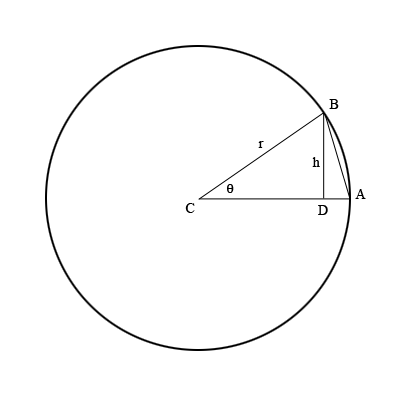SEARCH HOMEMath Central Quandaries & QueriesQuestion from Hari: why is area of a triangle drawn from centre of a circle i.e. radii forming the sides is 1/2 *r^2 sin theta where r is radiusHi,

I drew a diagram.The line segment $BD$ is perpendicular to the side $CA$ of the triangle so that $h,$ which is the length of $BD,$ is the height of the triangle. Write $h$ as a function of the angle measure $\theta.$

Penny* Registered trade mark of Imperial Oil Limited. Used under license.Math Central is supported by the University of Regina and the Imperial Oil Foundation.